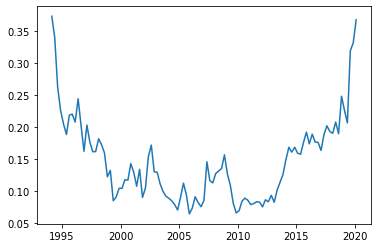# Python application 1: corporate leverage targets¶

The following regression of leverage ratios at quarter $t+1$ on the same at quarter $t$ $$\left(\frac{D}{V}\right)_{t+1} = \gamma \left(\frac{D}{V}\right)^* + (1-\gamma) \left(\frac{D}{V}\right)_{t} + \epsilon_{t+1}$$ should describe reasonably well the behavior of a corporation that partially adjusts its leverage ratio from where it currently is towards a long-term target $\left(\frac{D}{V}\right)^*,$ where $\gamma$ measures the speed of adjustment. Here $D$ is the value of all non-operating liabilities (see below for details) while $V$ is enterprise value. The current leverage ratio matters because adjusting one's leverage ratio either by issuing more debt or retiring some debt is costly and takes time. White noise $\epsilon$ simply reflects the fact that every one has a plan until they get punched in the mouth (Mike Tyson, 1987.)

Notice that if $\gamma=0$ the equation degenerates to $$\left(\frac{D}{V}\right)_{t+1} = \left(\frac{D}{V}\right)_{t} + \epsilon_{t+1}$$ which is known as a random walk. A corporation whose leverage ratio follows a random walk has no long-term target. Finding evidence of a long-term leverage target, therefore, is rejecting the hypothesis that $\gamma=0$.

Our main goal is to estimate $\gamma$ and $\left(\frac{D}{V}\right)^*.$ For that we'll need a few standard python modules. I usually import those blindly whether I expect to use them or not. I do most of the time anyway.

In :
import pandas as pd # pandas is excellent for creating and manipulating dataframes, R-style
import numpy as np # great for simulations, we may not use it here
import matplotlib.pyplot as plt #graphing module with matlab-like properties
%matplotlib inline
import requests # to make requests to webpages (like mine)
import statsmodels.api as sm # module full of standard statistical and econometric models, including OLS and time-series stuff
from IPython.display import Latex # to be able to display latex in python command cells


Next we import the data from my webpage and look at it a bit.

In :
df = pd.read_csv('http://erwan.marginalq.com/index_files/tea_files/IBMlast.csv')
type(df)
df.describe()

Out:
FF_CASH_ST FF_DEBT_ST FF_DEBT_LT FF_PFD_STK FF_MKT_VAL
count 105.000000 105.000000 105.000000 105.000000 105.000000
mean 9705.495238 9561.028571 22885.371429 103.466667 144308.221905
std 4781.393666 3300.757082 11446.970018 224.584491 48768.453063
min 3033.000000 4050.000000 9478.000000 0.000000 31853.300000
25% 6861.000000 6987.000000 14828.000000 0.000000 121991.000000
50% 9756.000000 9181.000000 18775.000000 0.000000 146355.000000
75% 11764.000000 12315.000000 28478.000000 247.000000 170714.000000
max 46408.000000 20543.000000 62391.000000 1091.000000 240675.000000
In :
df[0:4]

Out:
tic DATE FF_CASH_ST FF_DEBT_ST FF_DEBT_LT FF_PFD_STK FF_MKT_VAL
0 IBM 3/31/1994 8951 11942 14937 1091 31853.3
1 IBM 6/30/1994 8571 10357 14892 1091 34360.8
2 IBM 9/30/1994 10804 10240 14077 1091 40881.9
3 IBM 12/31/1994 10554 9570 12548 1081 43196.7

Next we create the variables we need. Notice the use of panda's shift operator to easily create a lagged variable.

In :
df['FF_PFD_STK']=df['FF_PFD_STK'].fillna(0) # note how we make sure that the NaN becomes zeros so as not to lose data
df['V']=df['FF_DEBT_ST']+df['FF_DEBT_LT']+df['FF_PFD_STK']+df['FF_MKT_VAL']-df['FF_CASH_ST'] # this is EV
df['D']=df['FF_DEBT_ST']+df['FF_DEBT_LT']+df['FF_PFD_STK']-df['FF_CASH_ST'] # this is leverage
df['Lev']=df['D']/df['V']
df['LevLag']=df.Lev.shift(1)


Next we plot leverage. Based on this it's obviously hard to tell whether IBM has a long-term tendency to return to some target. There seem to be three regimes: high leverage early on, low leverage in the middle, and high leverage again recently. Clearly, a regime-switching model would be a better way to represent IBM's leverage history. Still, for illustration, we will estimate our model as is.

In :
datenew=np.linspace(1,1,len(df['DATE'])) # we will build a date variable that makes for a prettier chart

for i in range(len(df['DATE'])):
datenew[i]=1994.125+ i*.25

plt.plot(datenew,df['Lev'])


Out:
[<matplotlib.lines.Line2D at 0x1aec6794e80>]Now we run our regression of leverage on lagged leverage.

In :
y=df.loc[1:,'Lev'] # note that this excludes the first row of data since lag is missing for that line
x=df.loc[1:,'LevLag']
x=sm.add_constant(x) # we run a standard OLS with constant
mod=sm.OLS(y,x)
res=mod.fit()
res.summary()

Out:
Dep. Variable: R-squared: Lev 0.845 OLS 0.843 Least Squares 555.9 Mon, 31 Aug 2020 4.43e-43 17:15:03 237.71 104 -471.4 102 -466.1 1 nonrobust
coef std err t P>|t| [0.025 0.975] 0.0121 0.006 1.966 0.052 -0.000 0.024 0.9164 0.039 23.577 0.000 0.839 0.994
 Omnibus: Durbin-Watson: 34.854 1.921 0 101.621 1.148 8.58e-23 7.264 16.3

Warnings:
 Standard Errors assume that the covariance matrix of the errors is correctly specified.

Now we need to convert those coefficients to the objects we are trying to estimate. Recall our model:$$\left(\frac{D}{V}\right)_{t+1} = \gamma \left(\frac{D}{V}\right)^* + (1-\gamma) \left(\frac{D}{V}\right)_{t} + \epsilon_{t+1}.$$ To get $\gamma$ we just need to do 1 - the coefficient on lag leverage, and then we can back out $\left(\frac{D}{V}\right)^*$ from the fact that the constant is an estimate of $\gamma \left(\frac{D}{V}\right)^*$. The python algebra below gives: $$\left[\gamma,\left(\frac{D}{V}\right)^*\right] \approx [0.084,0.145].$$

In :
gamma=1-res.params
DtoVstar=res.params/gamma
print('gamma estimates to %.3f or so' %gamma)
print('DtoVstar estimates to %.3f or so' %DtoVstar)

gamma estimates to 0.084 or so
DtoVstar estimates to 0.145 or so


Now we perform a DF test for H0: unit root. The default model in the module below is the one we ran, so no need to specify options, it's all baked in. We get a high p-value. We cannot reject the hypothesis that $\gamma=0$ in favor of the hypothesis with $\gamma>0$ with any sort of confidence, which is hardly surprising given the graph above.

In :
from statsmodels.tsa.stattools import adfuller

print('The p-value of H0 is %.5f' %adf_test)

The p-value of H0 is 0.22468


What if we exclude the latest leverage surge? After restricting the analysis to pre-2017 data, then we can reject the hypothesis that $\gamma=0$ with high confidence. This slicing data until we get the p-value we want is data-mining of the worst kind but this is consistent with what we saw on the chart above, namely the idea that IBM held their leverage in check until 2017 when something clearly changed.

In :
df['DATE'] = pd.to_datetime(df['DATE'])
slice = (df['DATE'] > '1994-1-1') & (df['DATE'] <= '2017-12-31')


The p-value of H0 is now 0.00024